Share filterDeveloped by in Education category

0/5.0 Ratings

10 - 50 Installs

0 Reviews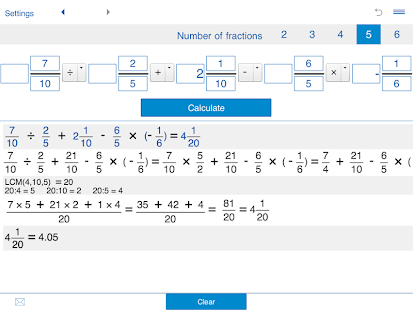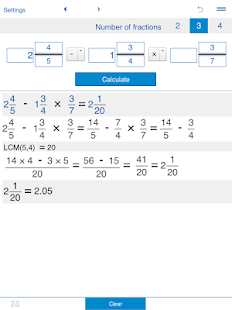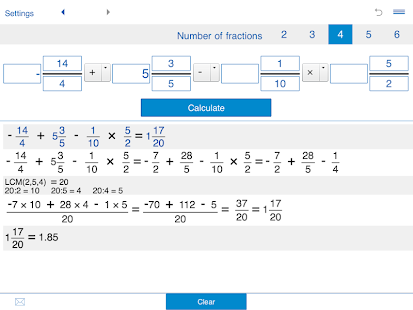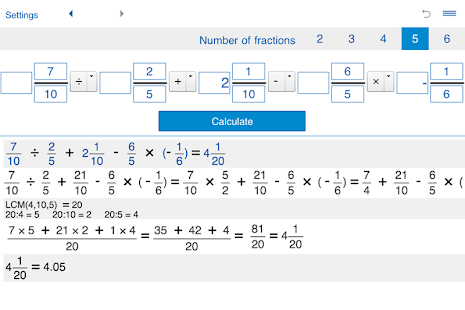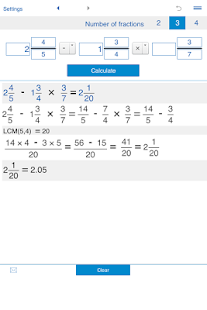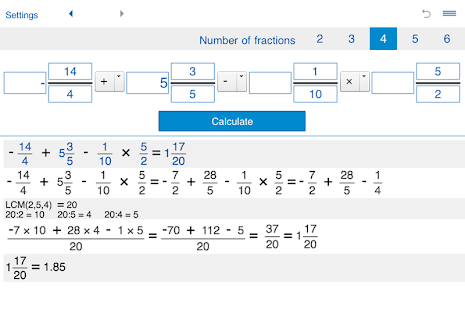### Description

Fraction calculator with steps to add, subtract, multiply, and divide fractions, whole and mixed numbers.

Features:

Fraction calculator for arithmetic operations with two, three, four, five, and six fractions: adding fractions, subtracting fractions, multiplying fractions, dividing fractions.

Fractions calculator helps you solve problems with fractions (proper and improper fractions), mixed numbers (mixed fractions), and whole numbers.

Addition and subtraction of fractions and mixed fractions with like and unlike denominators.

Multiplication and division of fractions and mixed numbers.

Finds the least common denominator.

Fraction solver shows the details of calculation.

History tape to view your recent calculations.

Back and forward buttons to check or recall recent calculations.

Sends results and history via email.

'Undo' for the Clear command.